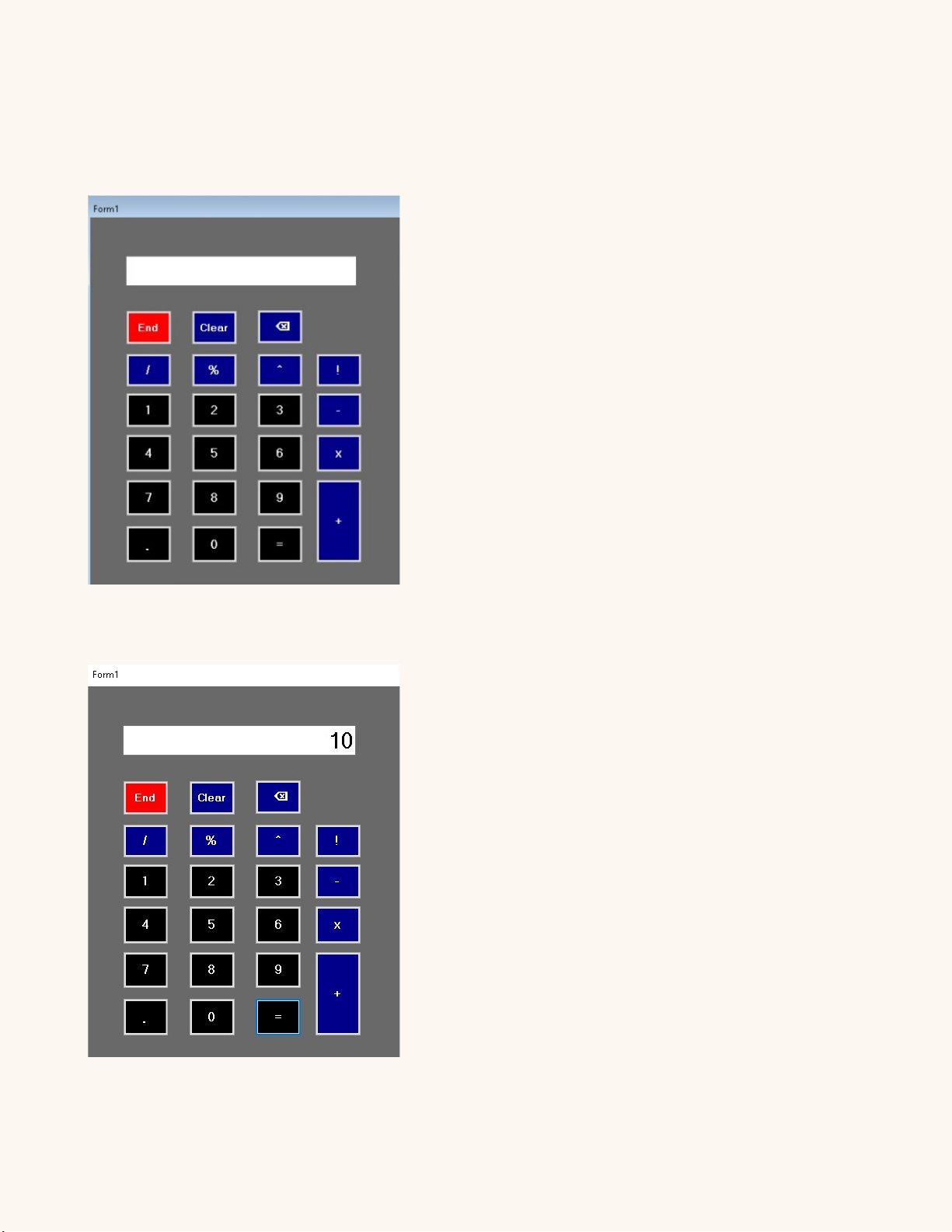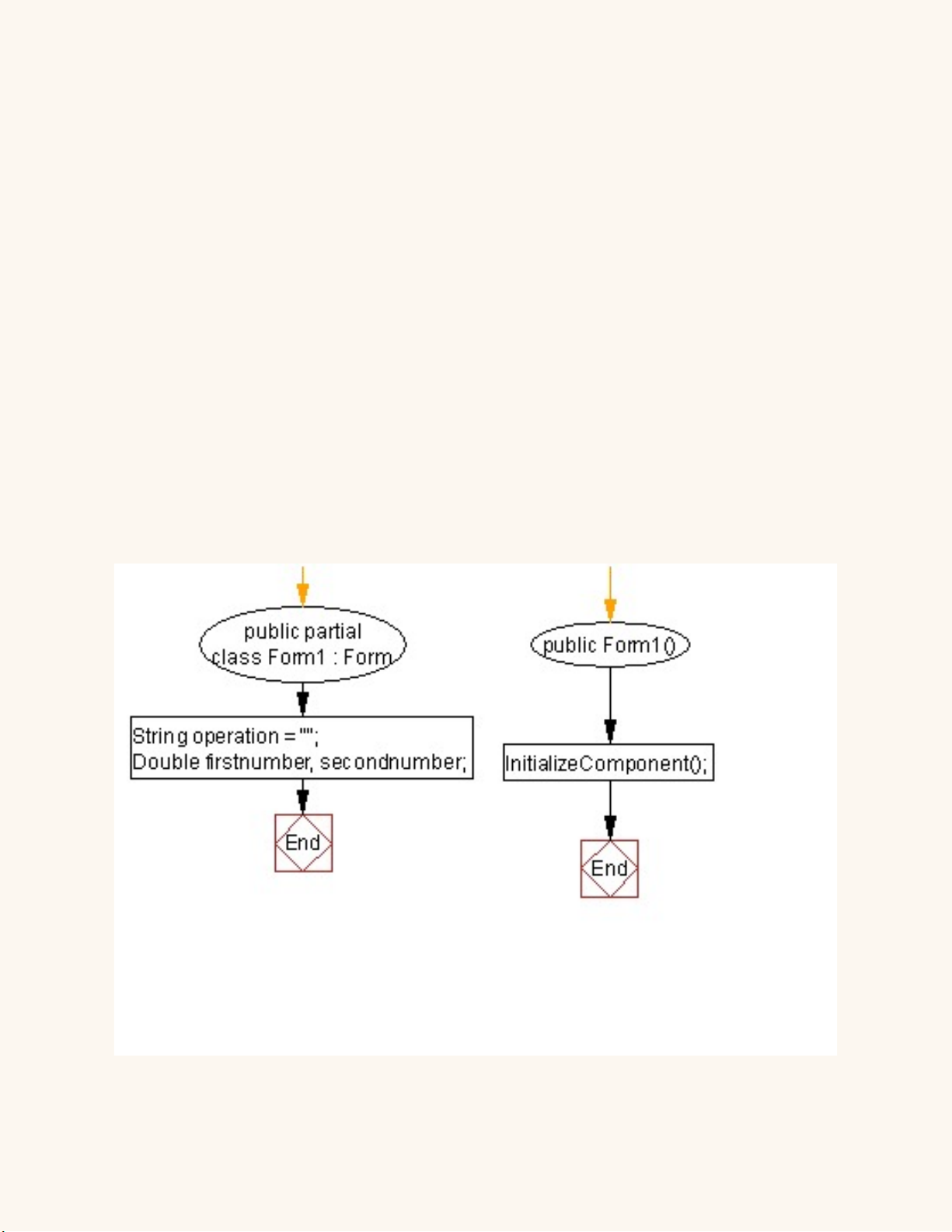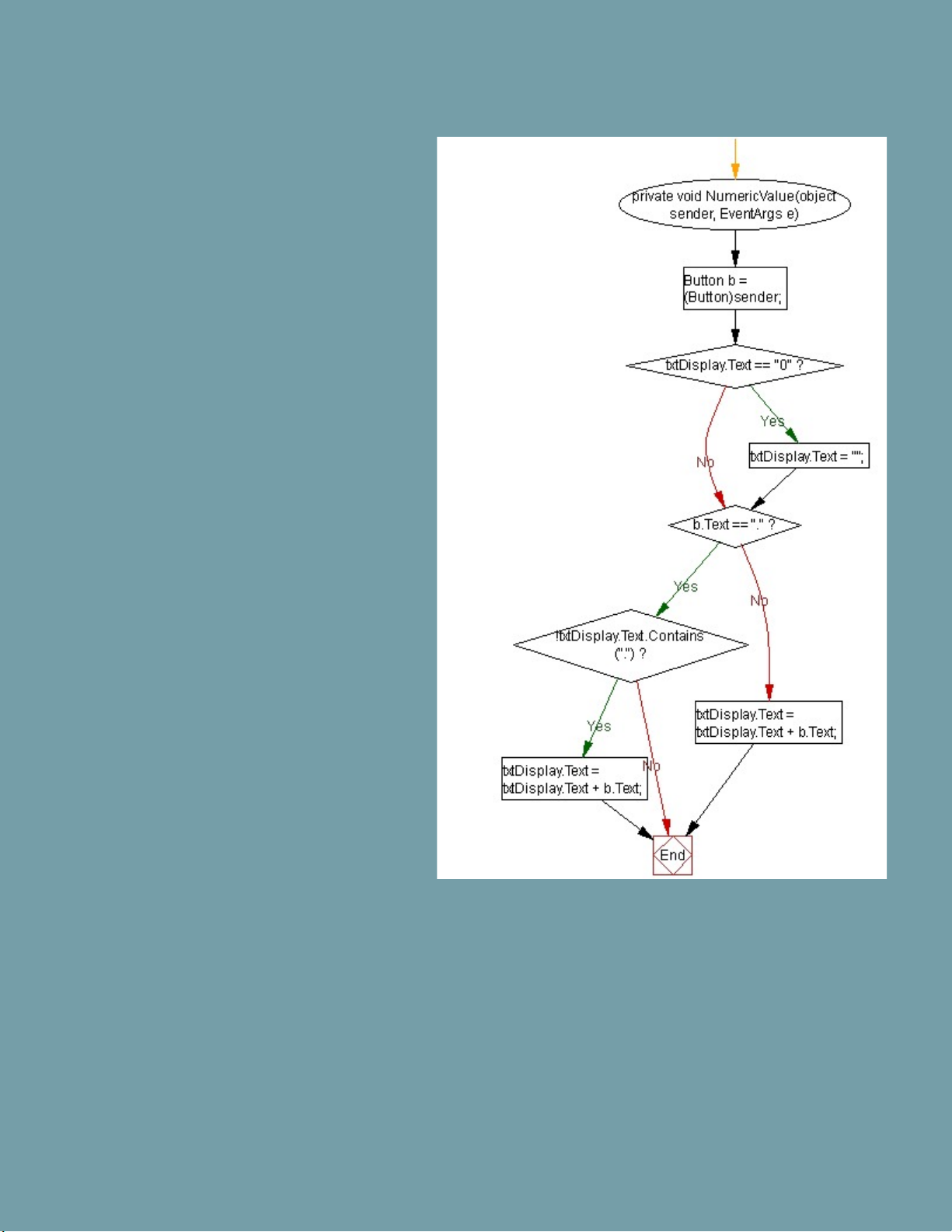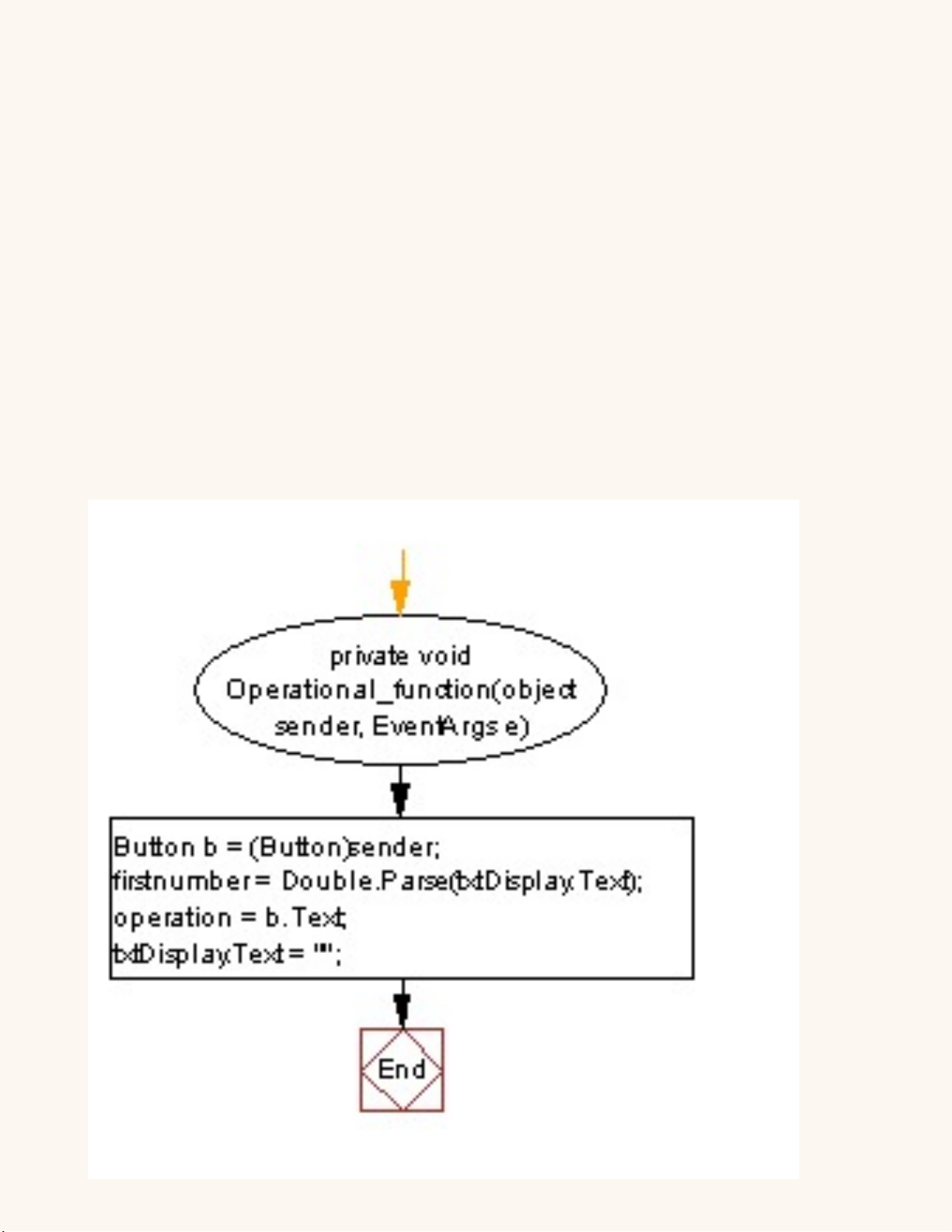!
Calculator Project
Allan 56090
Introduction
This Visual Studio 2019 C# calculator program will take two or one
number and one operator such as +, - , / , * , ^, %, ! and calculate
them.
Example;
1 + 1 = 2
8 - 4 = 4
12 / 2 = 6
2 * 4 = 8
10 ^ 1 = 10
50 % 100 = 50
5 ! = 120!
Photos
Photo1
Photo2
(after calculating two numbers)
5+5=10Code and Algorithm
Initially I declared variables, then took one or two numbers (ﬁrst number and second
number ) and use operations ( case & mathematical functions ) with the buttons to one
TextBox (text.Display) and calculate them (return result). This program uses switch,
function method.!
String operation = "";
"
Double ﬁrstnumber, secondnumber;
Means
Calculator will takes 2 numbers and does an operation with them.
InitializeComponent();
Is a method which is used to initialize your form.
It might be setting up things like the buttons, labels, event handlers,
and so on on your User Interface.Button b = (Button)sender;
Get the text value of the button
that was clicked
Sender refers to the object that
invoked the event that ﬁred the
event handler.Button b = (Button)sender;
ﬁrstnumber = Double.Parse(txtDisplay.Text);
operation = b.Text;
txtDisplay.Text = “";
ﬁrstnumber = Double.Parse(txtDisplay.Text);
Parsing to double
operation = b.Text;
if number includes point ( . )
txtDisplay.Text = “”;
Equals